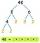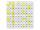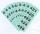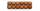# Prime Factorization Calculator

## 27 = 3 × 3 × 3 = 33

Divisors of number 27

This calculator will find all of the prime factors of a given number. Prime factorization or integer factorization of a number is the calculation of the set of prime numbers which multiply together to give the original integer. It is also known as prime decomposition.

The number 1 (one) is called a unit. It has no prime factors. When a number is a prime number (i.e., 2,3,5,7, 11...), the prime factorization is just a prime number. Other numbers are called composite numbers and have a minimum of two prime factors. To repeat, a prime number is a natural number that is divisible without a remainder by itself and by a unit, not by other natural numbers.

## Math problems:

27 = 3 × 3 × 3
198 = 2 × 32 × 11
1024 = 210
19823 = 43 × 461
30258037 = 30258037

## Word problems and examples involving prime numbers

• Prime factorsFactor the number 6600 into the product of prime numbers.
• Sum of two primesChristian Goldbach, a mathematician, found out that every even number greater than 2 can be expressed as a sum of two prime numbers. Write or express 2018 as a sum of two prime numbers.
• Prime factorsWrite 98 as a product of prime factors
• Prime divisorsFind 2/3 of the sum's ratio and the product of all prime divisors of the number 120.
• DecompositionMake decomposition using prime numbers of number 155. Result write as prime factors (all, even multiple)
• Primes 2Which prime numbers is number 2025 divisible?
• Unknown numberUnknown number is divisible by exactly three different primes. When we compare these primes in ascending order, the following applies: • Difference first and second prime number is half the difference between the third and second prime numbers. • The prod
• Six-digit primesFind all six-digit prime numbers that contain each one of digits 1,2,4,5,7, and 8 just once. How many are they?
• RaffleIn the pool is numbers from 1 to 115. What is the probability that a randomly selected number is not a prime number?
• Prime numberJan wrote any number from 1 to 20. What is the probability that he wrote the prime number?
• Amazing numberAn amazing number is name for such even number, the decomposition product of prime numbers has exactly three not necessarily different factors and the sum of all its divisors is equal to twice that number. Find all amazing numbers.
• John SmithJohn Smith is reading a 435-page novel. If he reads the same number of pages per day and the number is a prime number greater than 10, how many pages per day will he read?
• The ticketsThe tickets to the show cost some integer number greater than 1. Also, the sum of the price of the children's and adult tickets, as well as their product, was the power of the prime number. Find all possible ticket prices.
• Year 2018The product of the three positive numbers is 2018. What are the numbers?
• Lcm of three numbersWhat is the Lcm of 120 15 and 5
• Count of rootsHow many solutions has equation x. y = 7757 with two unknowns on the set of natural numbers?